# How does Yahoo Finance Calculate Beta?

Yahoo Finance gives beta values for stocks. But how are these values calculated? Find out how with a detailed step-by-step explanation.

This page on Yahoo Finance states that beta is calculated from monthly price for the previous 36 months, relative to the S&P 500.

This isn’t the whole story though. For important investment decisions, I want to reproduce the value using my own calculations, so that I understand how that number is arrived.

• Does it mean the 36 months ago from the current day? Or 36 months from the last complete month?
• To calculate beta, you need the returns. What’s used – the arithmetic returns or the log returns?
• Are the returns calculated using the close prices or the adjusted close prices?

Based on some detective work, I’ve concluded the following information and can successfully reproduce Yahoo Finance’s value of beta for any stock.

• You need 37 monthly prices (for your chosen ticker, and the S&P 500) from a start date to an end date.
• Returns will be calculate from months 2 to 37 (calculating the return for month 2 needs the price from month 1 – hence you need 37 prices to get 36 returns)
• Returns for month n should be calculated from this equation, where Rn is the return in month n, and Cn is the price in month n.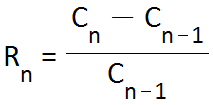• The monthly prices should be the close price on the first trading day of each month (note that this is not necessarily the first day of each month).
• The end date should be the first trading day of the month prior to the current month
• The start date should be the first trading day of the month 36 months prior to the end date
• Once you have the returns for your ticker and the S&P 500, beta is then calculated using Excel’s SLOPE() function.

Let’s put this theory into practice and attempt to reproduce Yahoo Finance’s beta, using raw monthly prices in Excel.

## Reproducing the Beta of Exxon Mobil as Given by Yahoo Finance

As of April 27th 2015, Yahoo Finance state that the beta of Exxon Mobil is 1.12. Follow these steps to reproduce this value (or download the complete spreadsheet at the bottom of this article).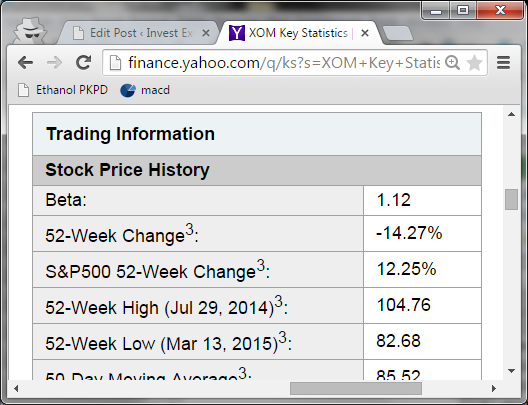Let’s say that we wanted to back-calculate this value.

Step 1: Download monthly close prices for XOM from here into a spreadsheet. Set the start date to “Mar 2 2012″ and the end date to Mar 30 2012”. This will give monthly prices from 1st March 2012 to 2nd March 2015

Step 2: Download monthly close prices for S&P 500 from here. Set the start date to “Mar 2 2012″ and the end date to Mar 30 2012”. Put these prices next to the column of XOM prices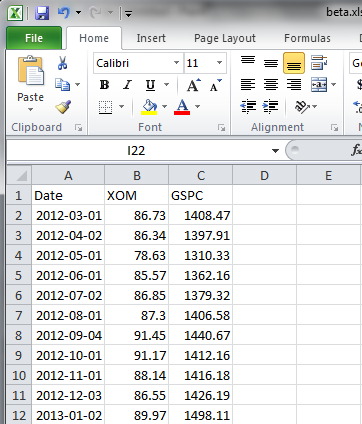You should have 37 monthly returns. For the spreadsheet I assembled, the returns went from Row 2 to Row 38

Step 3: Calculate the monthly returns for XOM and ^GSPC using the formula given above.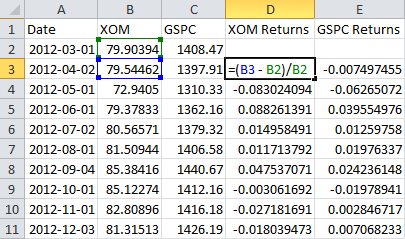For my spreadsheet, the returns went from Row 3 to Row 38. XOM’s returns are in Column D, while ^GSPC’s returns are in Column E.

Step 4: Calculate beta with Excel’s SLOPE() function, assuming that XOM is the “y” and ^GSPC is the “x”. For my spreadsheet, I entered =SLOPE(D3:D38,E3:E38)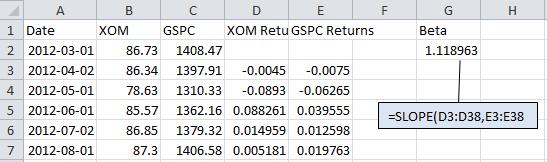The value returned is 1.119. This matches (to within rounding error) the value of 1.12 given on Yahoo Finance’s website on 27th April 2015.

I’ve reproduced beta values given on Yahoo Finance for many other stocks using this method.

Get Excel Spreadsheet to Reproduce Value of Beta Given by Yahoo Finance for XOM on April 27th 2015### 4 thoughts on “How does Yahoo Finance Calculate Beta?”

1. Do you really have all the spreadsheets locked?
For some reason, on my computer, I can open them all without any difficulty. I use excel 2013

• Not all of the spreadsheets are locked, a few are

2. Hi Samir,
Thanks so much for all this information, it’s really interesting. I have a silly quetion. When I try to download pricing from Yahoo it downloads the data into Excel in one column, recognized as text. I’ve tried to use “Text To Columns”, “Delimited”, “Comma”, “General”, which does break the numbers up into seperate columns, but the text is still not recognized as numbers.